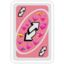MathematicsOpenStudy (anonymous):

The numerator of a fraction is 8 times the denominator. If 5 is subtracted from the numerator, and added to the denominator, the resulting fraction is equal to 11/2. Find the original fraction.OpenStudy (anonymous):

$n=8d\\ \frac{n-5}{d+5}=\frac{11}{2}$OpenStudy (anonymous):

So we can multiply both sides of the second equation by $$d+5$$, to get $$n-5=\frac{11}{2}(d+5)$$. So then $$n=\frac{11}2d+\frac{55}2+5,\ 2n=11d+60,\ 16d=11d+60,\ 5d=60$$... can you solve it from there?OpenStudy (anonymous):

This method might be ahead of what you've learned so far.OpenStudy (anonymous):

...I don't recognize it so that seems most likely 'x3OpenStudy (anonymous):

What method did you use to solve problems like this in class?OpenStudy (anonymous):

Online course with glitchy slideshows that don't explain things well... . _ .''OpenStudy (anonymous):

What course is it? Which grade, or what math level?OpenStudy (anonymous):

I'm in 12th but I failed part of Algebra freshman year and the just told me like...a month ago that I needed to make it up ~ _ ~''OpenStudy (anonymous):

Okay, this is something you should know then. This is basic algebra. You make a variable to represent the numerator, which I called $$n$$, and a variable to represent the denominator, which I called $$d$$, and then you write the word problem out using equations.

Latest QuestionsTyrion: Define love
6 minutes ago 6 Replies 2 Medalsogzec: Aristotle influenced medieval times. Describe the ideas inspired or borrowed from Aristotle.
23 minutes ago 0 Replies 0 Medalsdestiny14: Sarabeth used a box-and-whisker plot to summarize her race times in minutes. She didn't include her latest race time of 28 minutes.
30 minutes ago 0 Replies 0 MedalsBrookiecookie: Just need help with the last three Prompt: Now that you have finished reading the works in this unit, consider the ideals, values, and themes that early Ame
37 minutes ago 0 Replies 0 Medalskylieg123: An ice sculpture is melting from the heat. Assuming its height changes by negativ
1 hour ago 1 Reply 0 MedalsYuri: Oni was timing her 100 meter sprints. Her first five sprints were 12.568 seconds, 11.
1 hour ago 0 Replies 0 Medalsjc17: If you like to make logos and would like to make a logo for question cove this is what I'm using.
1 hour ago 9 Replies 1 Medalkayleigh344: Which expression can be used find 40% of 130?
1 hour ago 0 Replies 0 Medalsjc17: witch question cove logo do yall like?...
1 hour ago 10 Replies 1 Medalyesimfuny: How do you help yourself focused?
1 hour ago 9 Replies 1 Medal